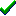# fg_trig()Win32DirectXFastgraph/Light

Prototype

void fg_trig (int angle, double *cosine, double *sine);

Sub fg_trig (ByVal angle As Long, cosine As Double, sine As Double)

procedure fg_trig (angle : integer; var cosine, sine : double);

Description

The fg_trig() function returns the floating point cosine and sine of a given angle. This function is called internally by Fastgraph's 3D functions and is not usually called directly by applications.

Parameters

angle is the angle, expressed in tenths of degrees.

cosine receives the cosine of the angle.

sine receives the sine of the angle.

Return value

none

Restrictions

none

Contents
Fastgraph Home Page

copyright 2001 Ted Gruber Software, Inc.